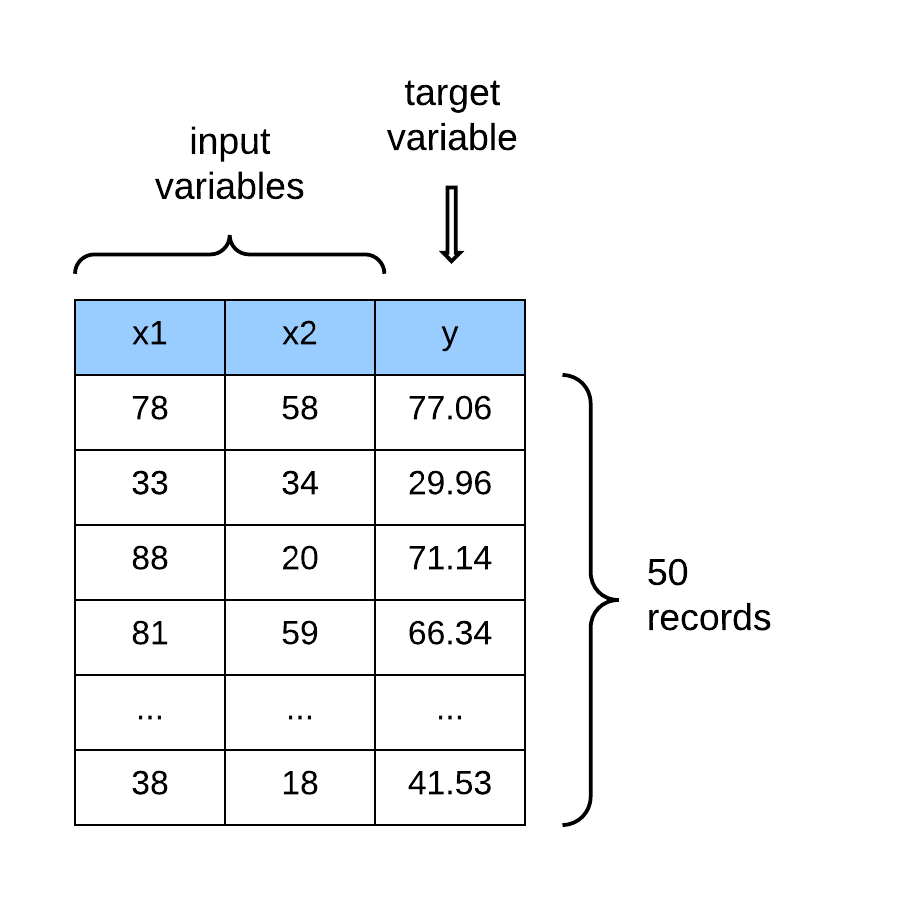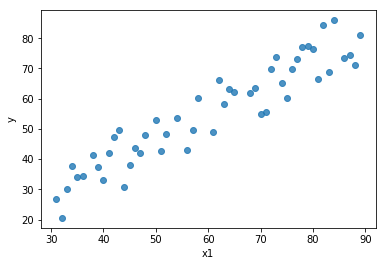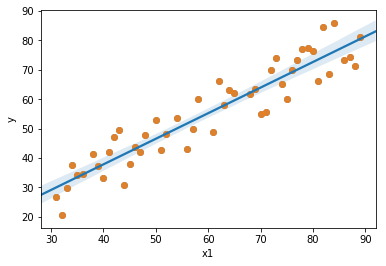Linear regression is used to predict real-valued outputs, and it assumes a linear dependence/ relationship between the variables of the dataset. Hence, it is a parametric algorithm. In linear regression, the prevailing assumption is that the output or the response variable (i.e., the unit that we want to predict) can be modeled as a linear combination of the input (or predictor) variables. A linear combination is the addition of a certain number of vectors scaled (or adjusted) by an arbitrary constant. A vector is a mathematical construct for representing a set of numbers.Figure 1: Dataset with real-valued outputs

In a linear regression model, every variable (or feature vector) is assigned a specific weight (or parameter). We say that a weight parameterizes each feature in the dataset. The weights (or parameters) in the dataset are adjusted to find the optimal value (or constant) that scales the features to optimally approximate the values of the target (or output variable). The linear regression model is formally represented as:

### A visual representation

To illustrate, the image below is a plot of the first feature and the target variable . We are plotting just one feature against the output variable because it is easier to visualize using a 2-D scatter plot.Figure 2: Scatter plot of x and y

So the goal of the linear model is to find a line that gives the best approximation or the best fit to the data points. When found, that line will look like the blue line in the image below.Figure 3: Scatter plot with the regression line

### Optimization for Machine Learning: Gradient Descent

Gradient descent is an optimization algorithm that is used to minimize a function (in this case we will be decreasing the cost or loss function of an algorithm). A cost function in machine learning is a measure that you want to minimize. We can also see the cost as the penalty you pay for having a bad or incorrect model approximation.

Gradient descent attempts to find an approximate solution or the global minimum of the function space by moving iteratively in step along the path of steepest descent until a terminating condition is reached that stops the loop or the algorithm converges.